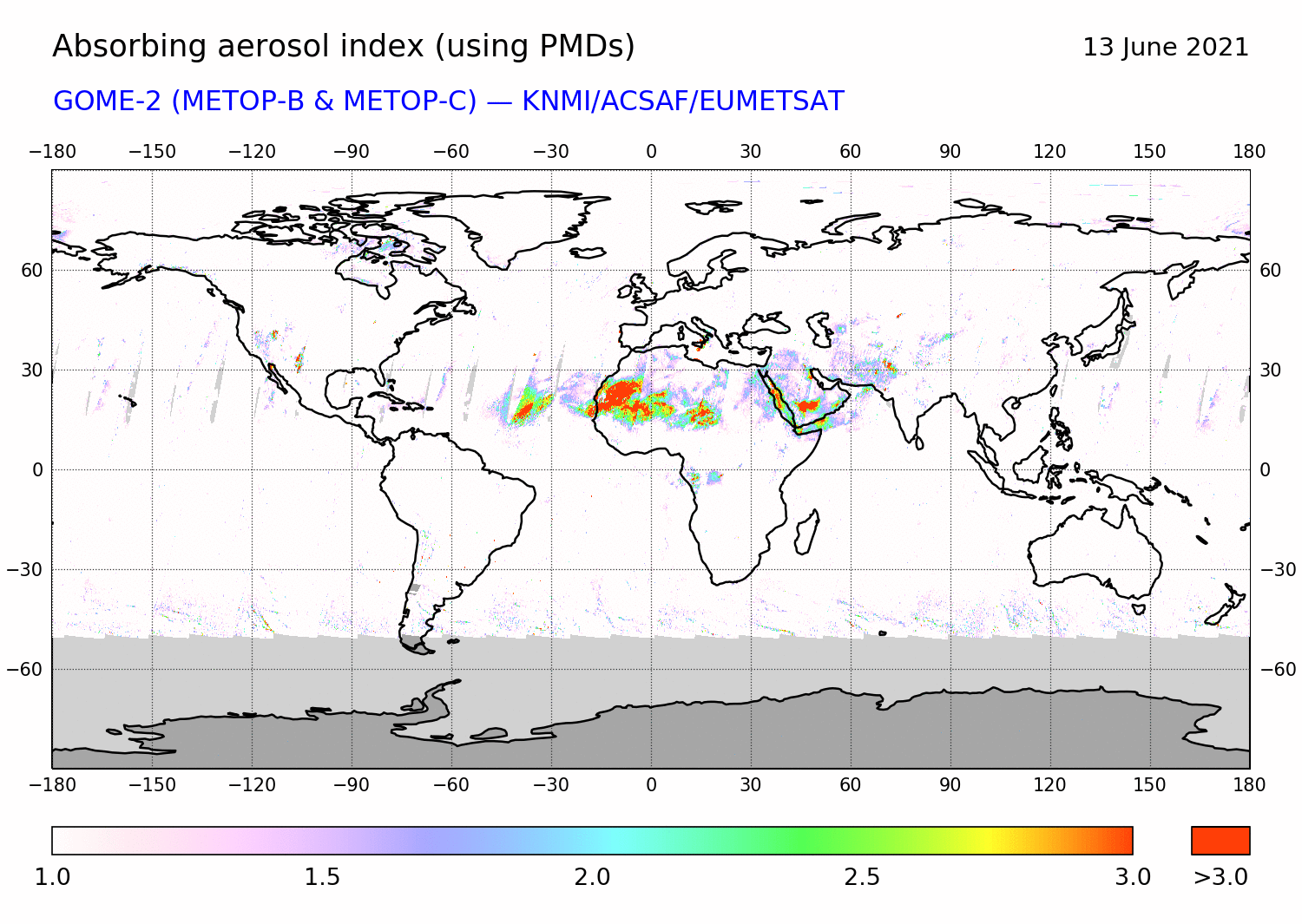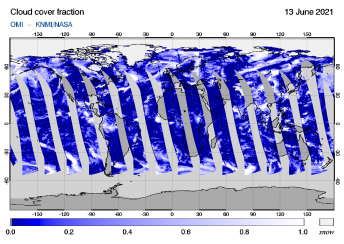SACS home > Nrt > near real-timeRoyal Belgian Institute for Space AeronomyNEAR REAL-TIME       NOTIFICATIONS       PRODUCTS### InfraRed

Instruments

GOME 2  OMI  OMPS  TROPOMI

IASI [A]  IASI [B]  AIRS

Time of observations
< day
< month
< year

### 13 June 2021

day >
month >
year >
 Select a date today    2002 2003 2004 2005 2006 2007 2008 2009 2010 2011 2012 2013 2014 2015 2016 2017 2018 2019 2020 2021 2022 Jan Feb Mar Apr May Jun Jul Aug Sep Oct Nov Dec 01 02 03 04 05 06 07 08 09 10 11 12 13 14 15 16 17 18 19 20 21 22 23 24 25 26 27 28 29 30 31    NRT Either click on a region in the map  to submit or select a region from        the list-menu and click 'submit' 000 == World view 001 == North pole 002 == South pole  ----------------- 101 == -150.0  60.0 102 == -120.0  60.0 103 ==  -90.0  60.0 104 ==  -60.0  60.0 105 ==  -30.0  60.0 106 ==    0.0  60.0 107 ==   30.0  60.0 108 ==   60.0  60.0 109 ==   90.0  60.0 110 ==  120.0  60.0 111 ==  150.0  60.0 112 ==  180.0  60.0  ----------------- 201 == -150.0  30.0 202 == -120.0  30.0 203 ==  -90.0  30.0 204 ==  -60.0  30.0 205 ==  -30.0  30.0 206 ==    0.0  30.0 207 ==   30.0  30.0 208 ==   60.0  30.0 209 ==   90.0  30.0 210 ==  120.0  30.0 211 ==  150.0  30.0 212 ==  180.0  30.0  ----------------- 301 == -150.0   0.0 302 == -120.0   0.0 303 ==  -90.0   0.0 304 ==  -60.0   0.0 305 ==  -30.0   0.0 306 ==    0.0   0.0 307 ==   30.0   0.0 308 ==   60.0   0.0 309 ==   90.0   0.0 310 ==  120.0   0.0 311 ==  150.0   0.0 312 ==  180.0   0.0  ----------------- 401 == -150.0 -30.0 402 == -120.0 -30.0 403 ==  -90.0 -30.0 404 ==  -60.0 -30.0 405 ==  -30.0 -30.0 406 ==    0.0 -30.0 407 ==   30.0 -30.0 408 ==   60.0 -30.0 409 ==   90.0 -30.0 410 ==  120.0 -30.0 411 ==  150.0 -30.0 412 ==  180.0 -30.0  ----------------- 501 == -150.0 -60.0 502 == -120.0 -60.0 503 ==  -90.0 -60.0 504 ==  -60.0 -60.0 505 ==  -30.0 -60.0 506 ==    0.0 -60.0 507 ==   30.0 -60.0 508 ==   60.0 -60.0 509 ==   90.0 -60.0 510 ==  120.0 -60.0 511 ==  150.0 -60.0 512 ==  180.0 -60.0 (region defined by the centre longitute and latitude)

## VOLCANOES in this region## Status of the NRT processingobs. of   SO2   SO2 height   Ash / AAI   Cloud

T
R
O
P
O
M
IG
O
M
E
-
2O
M
IO
M
P
SA
I
R
S